Scatter Diagram - Correlation & Regression, Business Mathematics & Statistics

# Scatter Diagram - Correlation & Regression, Business Mathematics & Statistics - Business Mathematics and Statistics - B Com

The Scatter Diagram Method is the simplest method to study the correlation between two variables wherein the values for each pair of a variable is plotted on a graph in the form of dots thereby obtaining as many points as the number of observations. Then by looking at the scatter of several points, the degree of correlation is ascertained.
The degree to which the variables are related to each other depends on the manner in which the points are scattered over the chart. The more the points plotted are scattered over the chart, the lesser is the degree of correlation between the variables. The more the points plotted are closer to the line, the higher is the degree of correlation. The degree of correlation is denoted by “r”.
The following types of scatter diagrams tell about the degree of correlation between variable X and variable Y.

1. Perfect Positive Correlation (r=+1): The correlation is said to be perfectly positive when all the points lie on the straight line rising from the lower left-hand corner to the upper right-hand corner.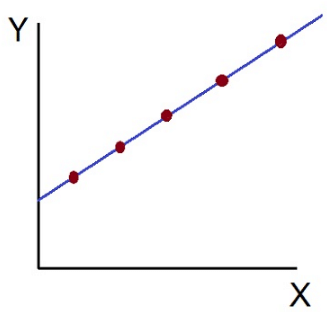2. Perfect Negative Correlation (r=-1): When all the points lie on a straight line falling from the upper left-hand corner to the lower right-hand corner, the variables are said to be negatively correlated.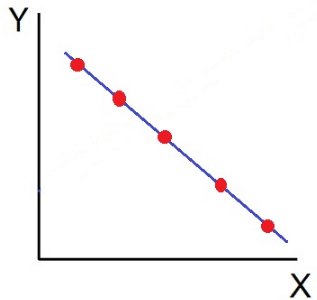3. High Degree of +Ve Correlation (r= + High): The degree of correlation is high when the points plotted fall under the narrow band and is said to be positive when these show the rising tendency from the lower left-hand corner to the upper right-hand corner.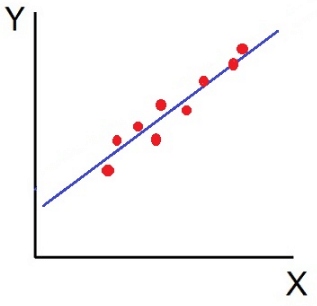4. High Degree of –Ve Correlation (r= – High): The degree of negative correlation is high when the point plotted fall in the narrow band and show the declining tendency from the upper left-hand corner to the lower right-hand corner.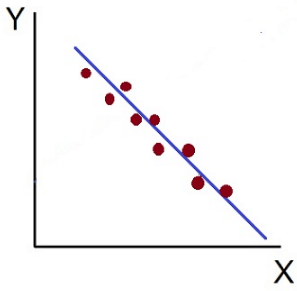5. Low degree of +Ve Correlation (r= + Low): The correlation between the variables is said to be low but positive when the points are highly scattered over the graph and show a rising tendency from the lower left-hand corner to the upper right-hand corner.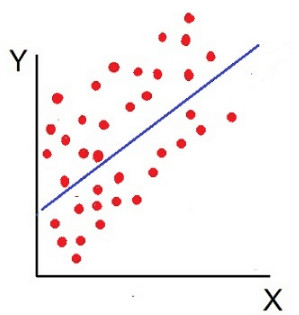6. Low Degree of –Ve Correlation (r= + Low): The degree of correlation is low and negative when the points are scattered over the graph and the show the falling tendency from the upper left-hand corner to the lower right-hand corner.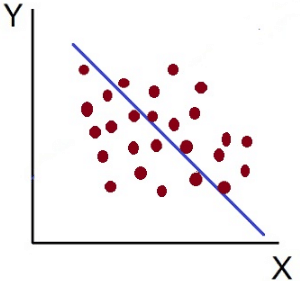7. No Correlation (r= 0): The variable is said to be unrelated when the points are haphazardly scattered over the graph and do not show any specific pattern. Here the correlation is absent and hence r = 0.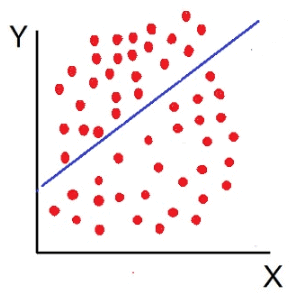Thus, the scatter diagram method is the simplest device to study the degree of relationship between the variables by plotting the dots for each pair of variable values given. The chart on which the dots are plotted is also called as a Dotogram.

The document Scatter Diagram - Correlation & Regression, Business Mathematics & Statistics | Business Mathematics and Statistics - B Com is a part of the B Com Course Business Mathematics and Statistics.
All you need of B Com at this link: B Com

115 videos|142 docs

## FAQs on Scatter Diagram - Correlation & Regression, Business Mathematics & Statistics - Business Mathematics and Statistics - B Com

 1. What is a scatter diagram and how is it used in business mathematics and statistics?Ans. A scatter diagram is a graphical tool used to display the relationship between two variables. It consists of a series of points plotted on a graph, with one variable represented on the x-axis and the other variable represented on the y-axis. Scatter diagrams are commonly used in business mathematics and statistics to analyze the correlation between variables and identify any patterns or trends.
 2. How can I determine the correlation between two variables using a scatter diagram?Ans. To determine the correlation between two variables using a scatter diagram, you need to examine the overall pattern of the points on the graph. If the points tend to form a straight line sloping upwards from left to right, it indicates a positive correlation. Conversely, if the points tend to form a straight line sloping downwards, it indicates a negative correlation. The closer the points are to the line, the stronger the correlation. If the points are scattered and do not form a clear pattern, it suggests no correlation or a weak correlation.
 3. Can a scatter diagram be used to predict future values of a variable?Ans. While a scatter diagram can provide insights into the relationship between two variables, it is not typically used for predicting future values. Scatter diagrams show the current relationship between variables, but they do not account for other factors that may influence future values. To make predictions, it is more appropriate to use regression analysis, which uses the data from the scatter diagram to create a mathematical model that can estimate future values based on the relationship between the variables.
 4. What is the difference between correlation and regression analysis in business mathematics and statistics?Ans. Correlation analysis is used to determine the strength and direction of the relationship between two variables. It measures how closely the variables are related, but it does not establish cause and effect. On the other hand, regression analysis is used to predict the value of one variable based on the value of another variable. It goes beyond correlation by establishing a mathematical relationship between the variables and allowing for the prediction of future values. Regression analysis can also identify the significance of independent variables in explaining the variation in the dependent variable.
 5. How can I interpret the results of a regression analysis based on a scatter diagram?Ans. When interpreting the results of a regression analysis based on a scatter diagram, it is important to consider the coefficient of determination (R-squared) and the regression equation. The R-squared value indicates the proportion of the variation in the dependent variable that can be explained by the independent variable(s). A higher R-squared value indicates a stronger relationship between the variables. The regression equation shows the mathematical relationship between the variables and can be used to predict the value of the dependent variable based on the values of the independent variable(s).

115 videos|142 docsExplore Courses for B Com examSignup to see your scores go up within 7 days! Learn & Practice with 1000+ FREE Notes, Videos & Tests.
10M+ students study on EduRev
Track your progress, build streaks, highlight & save important lessons and more!
Related Searches

,

,

,

,

,

,

,

,

,

,

,

,

,

,

,

,

,

,

,

,

,

,

,

,

;